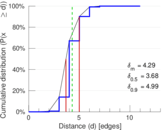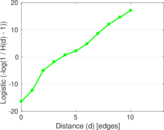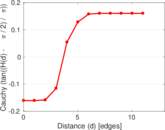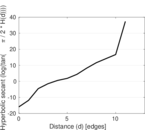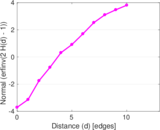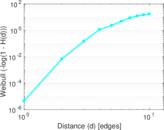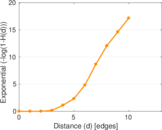# Orkut

This is the bipartite network of Orkut users and their group memberships.

 Code `OG` Internal name `orkut-groupmemberships` Name Orkut Data source http://socialnetworks.mpi-sws.org/data-imc2007.html AvailabilityDataset is available for download Consistency checkDataset passed all tests Category Affiliation network Node meaning User, group Edge meaning Membership Network formatBipartite, undirected Edge typeUnweighted, no multiple edges

## Statistics

 Size n = 11,514,053 Left size n1 = 2,783,196 Right size n2 = 8,730,857 Volume m = 327,037,487 Wedge count s = 2,528,039,357,274 Maximum degree dmax = 318,240 Maximum left degree d1max = 40,425 Maximum right degree d2max = 318,240 Average degree d = 56.806 7 Average left degree d1 = 117.504 Average right degree d2 = 37.457 7 Fill p = 1.345 85 × 10−5 Size of LCC N = 11,508,023 Diameter δ = 15 50-Percentile effective diameter δ0.5 = 3.678 65 90-Percentile effective diameter δ0.9 = 4.993 95 Mean distance δm = 4.290 25 Gini coefficient G = 0.874 319 Balanced inequality ratio P = 0.130 289 Left balanced inequality ratio P1 = 0.213 783 Right balanced inequality ratio P2 = 0.119 824 Relative edge distribution entropy Her = 0.853 523 Power law exponent γ = 1.494 86 Degree assortativity ρ = +0.000 575 114 Degree assortativity p-value pρ = 2.467 45 × 10−25 Spectral norm α = 3,037.36

## Plots

### Degree distribution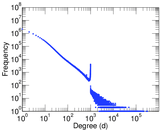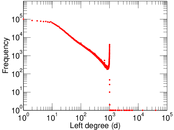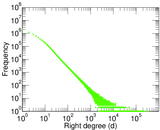### Cumulative degree distribution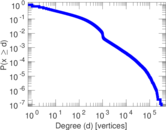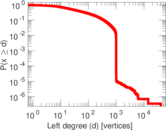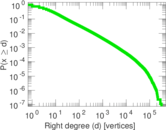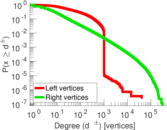### Lorenz curve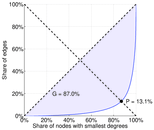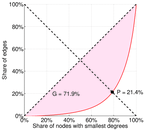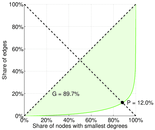### Spectral distribution of the adjacency matrix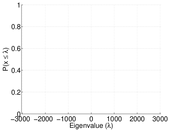### Spectral distribution of the normalized adjacency matrix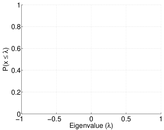### Spectral distribution of the Laplacian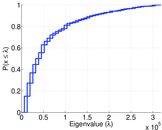### Spectral graph drawing based on the adjacency matrix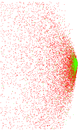### Spectral graph drawing based on the normalized adjacency matrix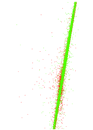### Degree assortativity### Hop distribution